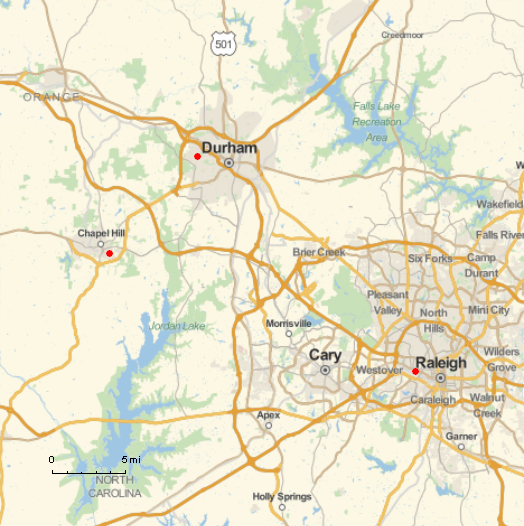# Spherical trig, Research Triangle, and Mathematica

This post will look at the triangle behind North Carolina’s Research Triangle using Mathematica’s geographic functions.

## Spherical triangles

A spherical triangle is a triangle drawn on the surface of a sphere. It has three vertices, given by points on the sphere, and three sides. The sides of the triangle are portions of great circles running between two vertices. A great circle is a circle of maximum radius, a circle with the same center as the sphere.

An interesting aspect of spherical geometry is that both the sides and angles of a spherical triangle are angles. Because the sides of a spherical triangle are arcs, they have angular measure, the angle formed by connecting each vertex to the center of the sphere. The arc length of a side is its angular measure times the radius of the sphere.

Denote the three vertices by AB, and C. Denote the side opposite A by a, etc. Denote the angles at AB, and C by α, β, and γ respectively.

## Research Triangle

Research Triangle is a (spherical!) triangle with vertices formed by Duke University, North Carolina State University, and University of North Carolina at Chapel Hill.

(That was the origin of the name, though it’s now applied more loosely to the general area around these three universities.)

We’ll take as our vertices

• A = UNC Chapel Hill (35.9046 N, 79.0468 W)
• B = Duke University in Durham (36.0011 N, 78.9389 W),
• C = NCSU in Raleigh (35.7872 N, 78.6705 W)## Mathematica

We’ll illustrate several features of Mathematica using the spherical triangle corresponding to Research Triangle.

### Map

The map above was produced with the following Mathematica code.

```    ptA = GeoPosition[{35.9046, -79.0468}]
ptB = GeoPosition[{36.0011, -78.9389}]
ptC = GeoPosition[{35.7872, -78.6705}]

GeoGraphics[{Red, PointSize[Large],
Point[ptA], Point[ptB], Point[ptC]},
GeoScaleBar -> "Imperial",
GeoRange -> 29000]
```

Note that longitude is in degrees east, so the longitudes above are negative.

### Distance

To find the distance between two locations, you can use the function `GeoDistance`. For example,

```    GeoDistance[ptA, ptB]
```

tells me that the distance between UNC and Duke is 8.99185 miles. I assume it displays miles by default based on my location in the US, though the `GeoRange` option above defaults to meters. You can make the system of units explicit. For example

```    GeoDistance[ptA, ptB, UnitSystem -> "Metric"]
```

returns 14.741 km.

If we want to find the length of the side c in degrees, we can use the Earth’s radius.

```    r = PlanetData["Earth", "Radius"]
c = GeoDistance[ptA, ptB] 360 / (2 Pi r)
```

This shows that c is 0.13014°. Calculating the other sides similarly shows a = 0.30504° and b = 0.32739°.

### Angles

Calling `GeoDirection[ptA, ptB]` returns 42.2432°, which says we need to head at an angle of about 42° to walk from UNC to Duke.

The code

`    GeoDirection[ptA, ptB] - GeoDirection[ptA, ptC]`

shows that the angle α is 68.6128°. (The code returns the negative of this angle because the angle is clockwise.) Similarly we find β = 87.9808° and γ = 23.4068.

The angles add up to 180° only because our triangle is small compared to the earth’s total area. The actual sum should be slightly more than 180°, but we’ve not retained enough precision to detect the difference. In general the “spherical excess,” i.e. the amount by which the sum of the angles exceed 180°, is proportional to the area of the triangle.

## Related posts